Reach Us+44-7480-724769
Contractions of 3-Dimensional Representations of the Lie Algebra sl(2) | OMICS International
Journal of Generalized Lie Theory and Applications
All submissions of the EM system will be redirected to Online Manuscript Submission System. Authors are requested to submit articles directly to Online Manuscript Submission System of respective journal.

Contractions of 3-Dimensional Representations of the Lie Algebra sl(2)

Department of Physics, Faculty of Nuclear Sciences and Physical Engineering, Czech Technical University, Brehova 7, 110 00 Prague, Czech Republic

*Corresponding Author:
Department of Physics,
Faculty of Nuclear Sciences and Physical Engineering,
Czech Technical University,
Brehova 7, 110 00 Prague,
Czech Republic
E-mail:
[email protected]

Received date: 22 September 2011, Revised date: 04 January 2012 Accepted date: 06 January 2012

Visit for more related articles at Journal of Generalized Lie Theory and Applications

Abstract

A theory of grading preserving contractions of representations of Lie algebras has been developed. In this theory, grading of the given Lie algebra is characterized by two sets of parameters satisfying a derived set of equations. Here we introduce a list of resulting 3-dimensional representations for the Z3-grading of the sl(2) Lie algebra.

Introduction

Contraction, the concept named by In¨on¨u and Wigner , is suitable for unification of theories, studying relations between symmetries, etc. The first papers about this concept were published in 1950’s by Segal . The next papers parallel to In¨on¨u and Wigner came from Saletan  and Doebner and Melsheimer . In this paper, contractions of representations are studied.

The problem is the following: starting with a given Lie algebra characterized by its commutation relations, a question arises: which Lie algebras can be obtained using graded contractions? This is a natural way of obtaining Lie algebras, starting with a fixed one. Moreover, for n-dimensional representations of the initial Lie algebra, using another tool of the subject, namely the contraction of representations, we can produce new representations of Lie algebras. Using both concepts simultaneously, we get representations of Lie algebras which arise as the graded contractions.

The contribution of this paper is the investigation of the Z3-graded contraction of 3-dimensional representation of the complex Lie algebra sl(2). In Sections 2 and 3, graded contractions are defined and basic features of sl(2) are described. We study which Lie algebras can be obtained by contractions of sl(2). In Section 4, we introduce the definition of graded contractions of representations of Lie algebras. It is a tool for construction of new Lie algebras from a given Lie algebra. Section 5 describes all contractions of 3-dimensional representations of Z3-graded sl(2). For this purpose, results published in [1,4] will be applied.

Graded contractions of Lie algebras

Graded contractions of Lie algebras are a tool for the production of new Lie algebras from the original Lie algebra. In this section, we introduce the definition of this term.

Definition 1. Let n ∈ N and let g be a Lie algebra. A grading is the decomposition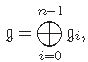where the commutators satisfy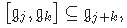Definition 2. The graded contraction of the given Lie algebra g is the Lie algebra gε which is isomorphic to the given Lie algebra g as a linear space, and with commutation relations preserving the grading [gj ,gk] ⊆ gj+k: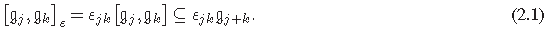The contraction parameters εjk should satisfy the equations

εjkεj+k,m = εkmεj,k+m. (2.2)

We define the contraction matrix ε as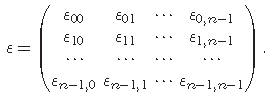From (2.1), it follows that εjk = εkj (i.e., the matrix is symmetric). Taking into account (2.2), we get in the case of Z3-graded contractions the equations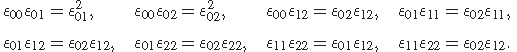Then we have (up to equivalence of matrices corresponding to the change of bases of the subspaces g1 and g2) these possible forms of the contraction matrix :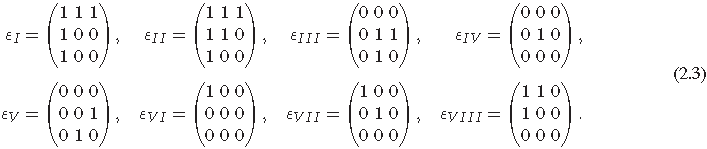The case of sl(2)

The Lie algebra sl(2) is one of the main kinds of the simple Lie algebras—sl(n+1) ≡ An. The dimension is given by the number of its generators, and it is n2 +2n (i.e. the dimension of sl(2) is 3). We denote its generators h, e,f. They satisfy the commutation relations

[h,e] = 2e, [h,f] = −2f, [e,f] = h. (3.1)

In the case of the Z3-grading, we have the decomposition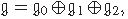where

g0 = {h}lin, g1 = {e}lin, g2 = {f}lin. (3.2)

We apply the matrices from (2.3) on (2.1) and use the notation of (3.2), then we have only 4 distinct possibilities how the commutation relations (3.1) can be changed:

(1) [h,e] = 2e, [h,f] =−2f, [e,f] = 0 which corresponds to the Euclidean algebra e(2) coinciding with the matrices εI and εII ,

(2) [h,e] = 0, [h,f] = 0, [e,f] = h corresponding to the Heisenberg algebra h coinciding with the matrices εIII and εV ,

(3) [h,e] = 0, [h,f] = 0, [e,f] = 0 corresponding to the Abelian algebra c coinciding with the matrices εIV , εV I and εV II,

(4) [h,e] = 2e, [h,f] = 0, [e,f] = 0 corresponding to the algebra which we denote l, coinciding with the matrix εV III .

Graded contractions of representations of sl(2)

Starting with the definition of graded contractions of representations, we find different sets of equivalent contractions of Lie algebras and representations for the Z3-grading, and we ask which Lie algebras correspond to the representations given by the Z3-graded contractions of the 3-dimensional representations of the Lie algebra sl(2).

Definition 3. Let g be a Zp-graded Lie algebra, where p ∈ N, and let V be a g-module. Then assume that the action of Zp-graded g on V respects the grading on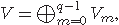that is

0 = T(gj)ψ Vm ⊆ Vj+m, (4.1)

where Vj , j = 0, . . . , q−1, defines the corresponding Zq-grading of V and the addition of indices is modulo q. If one denotes the contracted action T(g) on V as

T(gj) ψ ·Vm ⊆ ψjmT(gj) Vm, (4.2)

where ψjm are the contraction parameters, then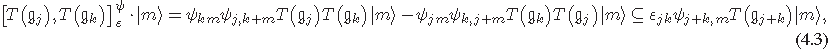where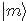, m = 0, . . . , q−1, are the base vectors of the subspace Vm, ε and εjk denote the contraction of g and the corresponding parameters. The relations (4.3) are definitely satisfied when

εjkψj+k,m = ψkmψj,k+m = ψjmψk,j+m. (4.4)

One uses the notation Vψ for V considered as graded T(gε)-module, where ψ satisfies (4.4). Vψ is then called the contraction of the representation V with respect to the contraction ε.

We write the contraction parameters into the matrix as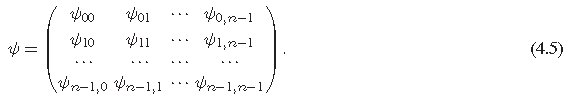In the case of contractions of Z3-graded representations of Z3-graded Lie algebra, the system of equations (4.4) gives the following relations: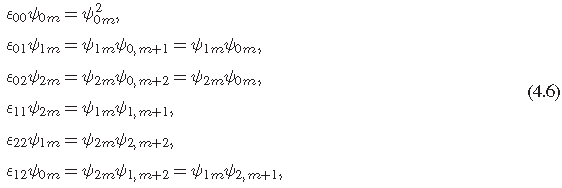where m ∈ {0,1,2}. From this it follows that (4.6) leads to the system of 18 equations, where for the simplification we suppose that either ψjk =0 or ψjk =1 for arbitrary j,k. Now we denote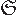the set of the right-hand sides of (4.6). We divide this set into six parts, each containing a triplet of numbers corresponding to a concrete set of equations in (4.6):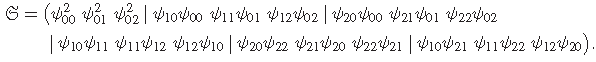There is a possibility of the cyclic permutation of columns of the contraction matrix ψ and each of these permutations corresponds to the cyclic permutation of numbers in each of the mentioned triplets. Starting with a given contraction matrix ψ, we denote ψ', respectively ψ", the contractions matrices, where all the columns are shifted one position to right, respectively to left.

The possible forms of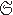depend on a pair of contraction matrices (ε,ψ), where ε was assigned in (2.3) and both ε and ψ can be found in the list of possible contractions of representations published in  which is also written in the appendix of this paper. We can distinguish these 12 possibilities: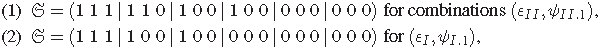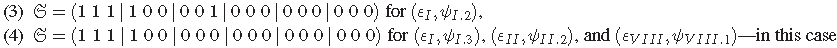all the matrices ψ have the same form,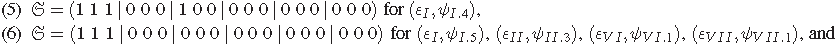V IIIV III.2) with the same form of the matrices ψ,

(7)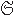=(1 1 0|1 0 0|0 0 0|0 0 0|0 0 0|0 0 0) for combinations with the same form of the matrices ψ (εII.6), (εIIII.4), and (εV IIIV III.3),

(8)= (1 1 0 | 0 0 0 | 0 1 0 | 0 0 0 | 0 0 0 | 0 0 0) for (εII.7),

(9)=(1 1 0|0 0 0|0 0 0|0 0 0|0 0 0|0 0 0) for combinations with the same form of the matrices ψ (εII.8), (εIIII.5), (εV IV I.2), (εV IIV II.3), and (εV IIIV III.4),

(8)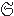= (1 1 0 | 0 0 0 | 0 1 0 | 0 0 0 | 0 0 0 | 0 0 0) for (εII.7),

(9)=(1 1 0|0 0 0|0 0 0|0 0 0|0 0 0|0 0 0) for combinations with the same form of the matrices ψ (εII.8), (εIIII.5), (εV IV I.2), (εV IIV II.3), and (εV IIIV III.4),

(10)= (1 0 0 | 0 0 0 | 0 0 0 | 0 0 0 | 0 0 0 | 0 0 0); here we have 3 distinct sets of pairs with the same matrices ψ: – (εII.9), (εIIII.6), (εV I,ψV I.5), (εV II,ψV II.4), and (εV III,ψV III.5), – (εV I,ψV I.3) and (εV IIV II.2), – (εV IV I.4) and (εV IIIV III.6),

(11)= (0 0 0 | 0 0 0 | 0 0 0 | 0 1 0 | 0 0 0 | 0 0 0) for combinations with the same form of the matrices ψ: (εIIIIII.1), (εIVIV.1), and (εV IIV II.5),

(12)= (0 0 0 | 0 0 0 | 0 0 0 | 0 0 0 | 0 0 0 | 0 0 0); we have 6 distinct sets of pairs of matrices, each of them containing just 1 form of the matrix ψ:
– (εIIIIII.2) and (εIVIV.2),
– (εVV.1), – (εVV.2) and (εV IV I.6),
– (εVV.3), (εV IV I.8), and (εV IIV II.6),
– (εVV.4), (εV IV I.9), and (εV IIIV III.7),
– (εV IV I.7).

Now we investigate how the contractions in the previous list transform the 3-dimensional representations of sl(2). First, we study the cases which coincide with the representations of the Abelian algebra c. These cases immediately provide the solution, because the Abelian algebra cannot be decomposed into anything simpler. In Section 3, we see that the Abelian algebra c coincides with the contraction matrices εIV , εV I, and εV II. This means that we know the solution for the cases (6), (9), (10), (11), and (12) in the previous list.

In order to study the other cases, we start with the 3-dimensional representation of sl(2). All irreducible 3- dimensional representations of sl(2) are isomorphic with the representation T given by matrices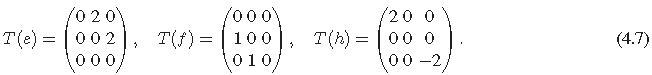Now we consider the remaining cases using this representation T, the corresponding contraction matrices ψ from  introduced in the list of all possible contractions of 3-dimensional representations and the contraction matrices ψ ', ψ '" arising from ψ by the cyclic permutation of columns. According to the properties of commutation relations of the matrices corresponding to the resulting representations, we distinguish representations corresponding to Lie algebras e(2), c, l, and l', where l' and l differ in exchanging the commutation relations for e and f: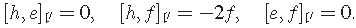We explain the procedure on the first case, corresponding to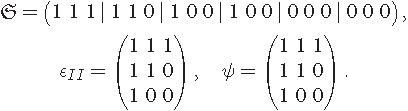First, we have to find the grading of the g-module corresponding to (4.1). It appears that the only suitable grading has the form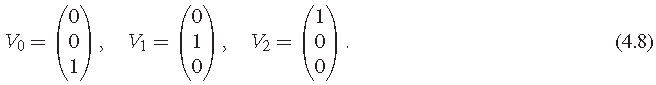Now we take the representations T from (4.7) and we multiply the columns of the particular matrices (from right to left in agreement with the grading (4.8)) by the elements of the rows of the matrix ψ: the first row in ψ corresponds to T(h), the second row corresponds to T(e), the third row corresponds to T(f). By this way, we get a new representation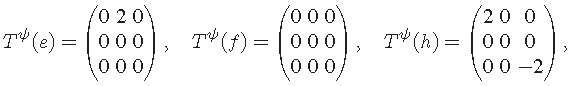its commutation relations correspond to the Lie algebra l. Next, we compose the matrices ψ ' and ψ " which arise by the permutation of the columns of ψ and get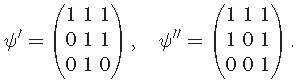Now we repeat the same procedure as we did for ψ and get the matrices corresponding to representations of e(2) for both ψ ' and ψ".

The results for all the cases are listed in Table 1, where for each case in the previous list, the resulting representation is written.

Conclusion

The presented results of the contractions of 3-dimensional representations of sl(2) are applicable in some mathematical areas.

One of them is the area of universal enveloping algebras. The problem, depicted in the following diagram, is the following: we investigate if an adjoint representation ρ represented in a given universal enveloping algebra U(g) associated with a Lie algebra g can be simplified by a contraction ψ to the corresponding adjoint representation ρε represented in another universal enveloping algebra U(gε) associated with a Lie algebra g which is a contraction of g. It can be shown that in the case g ≡ sl(2), this task is solvable for the 3-dimensional representations, but for the higher dimensions it is unfeasible. The main problem consists in the need of the same base for the matrices of both representations. The form of the matrices corresponding to the representation of the contracted g in the given base is too difficult to be obtained with the help of the contraction.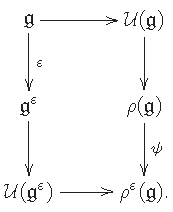Acknowledgments

The support by the Ministry of Education of Czech Republic (project MSM6840770039) is acknowledged as well as support by the Grant Agency of the Czech Technical University in Prague, Grant no. SGS11/132/OHK4/2T/14. The authors are grateful to the referees for constructive remarks which helped to improve the presentation.

References

Select your language of interest to view the total content in your interested language

Article Usage

• Total views: 12070
• [From(publication date):
September-2012 - Oct 23, 2019]
• Breakdown by view type
• HTML page views : 8270Can't read the image? click here to refresh